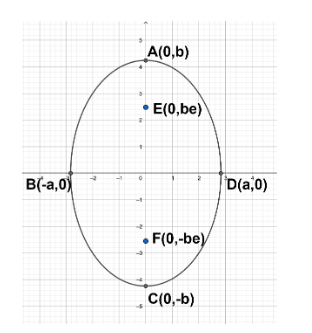QuestionAnswers

# If $x=2\left( \cos \text{t}-\sin \text{t} \right)$; $y=3\left( \cos \text{t}+\sin \text{t} \right)$ represents a conic, its foci are:a)$(\pm \sqrt{10},0)$b)$(\pm \sqrt{13},0)$c)$(0,\pm \sqrt{13})$d)$(0,\pm \sqrt{10})$Verified
131.4k+ views
Hint: Firstly, try to find the conic represented by the given function by simplifying the equations using mathematical identities and get an equation in terms of x and y.
Then convert the equations into a standard form of any conic section (i.e. ellipse, parabola, or hyperbola).
Circle: ${{\left( x-h \right)}^{2}}+{{\left( y-k \right)}^{2}}={{r}^{2}}$
Parabola: \left\{ \begin{align} & y=\dfrac{{{\left( x-h \right)}^{2}}}{4a}+k \\ & x=\dfrac{{{\left( y-k \right)}^{2}}}{4a}+h \\ \end{align} \right\}
Ellipse: $\dfrac{{{\left( x-h \right)}^{2}}}{{{a}^{2}}}+\dfrac{{{\left( y-k \right)}^{2}}}{{{b}^{2}}}=1$
Hyperbola: \left\{ \begin{align} & \text{for a b : }\dfrac{{{\left( x-h \right)}^{2}}}{{{a}^{2}}}-\dfrac{{{\left( y-k \right)}^{2}}}{{{b}^{2}}}=1 \\ & \text{for a b : }\dfrac{{{\left( y-k \right)}^{2}}}{{{b}^{2}}}-\dfrac{{{\left( x-h \right)}^{2}}}{{{a}^{2}}}=1 \\ \end{align} \right\}\left\{ \begin{align} & \text{for a b : }\dfrac{{{\left( x-h \right)}^{2}}}{{{a}^{2}}}-\dfrac{{{\left( y-k \right)}^{2}}}{{{b}^{2}}}=1 \\ & \text{for a b : }\dfrac{{{\left( y-k \right)}^{2}}}{{{b}^{2}}}-\dfrac{{{\left( x-h \right)}^{2}}}{{{a}^{2}}}=1 \\ \end{align} \right\}
After getting the equation of the conic section, find the foci for the required conic section.

Consider the given functions:
\begin{align} & x=2\left( \cos \text{t}-\sin \text{t} \right)......(1) \\ & y=3\left( \cos \text{t}+\sin \text{t} \right)......(2) \\ \end{align}
Squaring the both sides of equation (1) & (2),
By applying the identities:\left[ \begin{align} & \because {{\left( a+b \right)}^{2}}={{a}^{2}}+{{b}^{2}}+2ab \\ & \because {{\left( a-b \right)}^{2}}={{a}^{2}}+{{b}^{2}}-2ab \\ \end{align} \right]\left[ \begin{align} & \because {{\left( a+b \right)}^{2}}={{a}^{2}}+{{b}^{2}}+2ab \\ & \because {{\left( a-b \right)}^{2}}={{a}^{2}}+{{b}^{2}}-2ab \\ \end{align} \right]
we get:
\begin{align} & {{x}^{2}}=4\left( {{\cos }^{2}}\text{t +}{{\sin }^{2}}\text{t}-2\sin \text{t}\text{.}\cos \text{t} \right)......(3) \\ & {{y}^{2}}=9\left( {{\cos }^{2}}\text{t}+{{\sin }^{2}}\text{t }+\text{ }2\sin \text{t}\text{.}\cos \text{t} \right)......(4) \\ \end{align}

We can also write above equations as:
\begin{align} & \dfrac{{{x}^{2}}}{4}=\left( {{\cos }^{2}}\text{t +}{{\sin }^{2}}\text{t}-2\sin \text{t}\text{.}\cos \text{t} \right)......(5) \\ & \dfrac{{{y}^{2}}}{9}=\left( {{\cos }^{2}}\text{t}+{{\sin }^{2}}\text{t }+\text{ }2\sin \text{t}\text{.}\cos \text{t} \right)......(4) \\ \end{align}
Add both the equations (5) & (6), we get:
\begin{align} & \dfrac{{{x}^{2}}}{4}+\dfrac{{{y}^{2}}}{9}=\left( {{\cos }^{2}}\text{t +}{{\sin }^{2}}\text{t}-2\sin \text{t}\text{.}\cos \text{t} \right) \\ & +\left( {{\cos }^{2}}\text{t}+{{\sin }^{2}}\text{t }+\text{ }2\sin \text{t}\text{.}\cos \text{t} \right) \\ & ={{\left( {{\cos }^{2}}\text{t +}{{\sin }^{2}}\text{t} \right)}^{2}}.......(7) \end{align}
Since $\left( {{\cos }^{2}}\text{t +}{{\sin }^{2}}\text{t} \right)=1$, equation (7) becomes:
$\dfrac{{{x}^{2}}}{4}+\dfrac{{{y}^{2}}}{9}=2......(8)$
Equation (8) can also be written as: $\dfrac{{{x}^{2}}}{8}+\dfrac{{{y}^{2}}}{18}=1......(9)$

Compare equation (8) with the standard form of various conic sections.
We get to know that equation (8) represents ellipse whose standard form of equation is $\dfrac{{{\left( x-h \right)}^{2}}}{{{a}^{2}}}+\dfrac{{{\left( y-k \right)}^{2}}}{{{b}^{2}}}=1$
Hence, $\dfrac{{{x}^{2}}}{8}+\dfrac{{{y}^{2}}}{18}=1$ is an ellipse, where h=k=0; a= $\sqrt{8}$ and b= $\sqrt{18}$ .
Since a < b, the major axis of ellipse is parallel to the y-axis.
The ellipse represented by equation (8) is shown with the help of a diagram below. Here BD is the major axis and AC is the minor axis.
The foci of the ellipse lies on the major axis which is the y-axis itself. Those are represented by E $\left( 0,be \right)$ and F $\left( 0,-be \right)$ where ‘e’ is the eccentricity of the ellipse.Now to find the foci of the ellipse, we need to calculate the eccentricity (e) of the ellipse.
i.e. for ellipse $\dfrac{{{\left( x-h \right)}^{2}}}{{{a}^{2}}}+\dfrac{{{\left( y-k \right)}^{2}}}{{{b}^{2}}}=1$;
If a < b, then $e=\sqrt{1-\dfrac{{{b}^{2}}}{{{a}^{2}}}}$ $e=\sqrt{1-\dfrac{{{a}^{2}}}{{{b}^{2}}}}$
If a > b, then $e=\sqrt{1-\dfrac{{{b}^{2}}}{{{a}^{2}}}}$
Therefore, eccentricity of the given ellipse $\dfrac{{{x}^{2}}}{8}+\dfrac{{{y}^{2}}}{18}=1$ is $e=\sqrt{1-\dfrac{8}{18}}$
$e=\sqrt{\dfrac{10}{18}}$
$e=\sqrt{\dfrac{2\times 5}{2\times 9}}$
$e=\dfrac{\sqrt{5}}{3}$
So focii of given ellipse are $\left( 0,\sqrt{18}\times \dfrac{\sqrt{5}}{3} \right)$ and $\left( 0,-\sqrt{18}\times \dfrac{\sqrt{5}}{3} \right)$
i.e. $(0,+\sqrt{10})$and $(0,-\sqrt{10})$
So, the correct answer is “Option D”.

Note: Be careful while identifying the major axis of ellipse while considering the values of a and b because it might change the value of eccentricity and therefore foci would lie parallel to the x-axis in another case (i.e. a > b).
Suppose we subtract the square of both the equations instead of adding them to simplify and get an equation in terms of x and y.